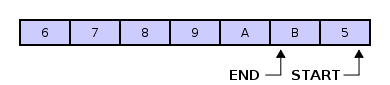Circular buffers are awesome! For those who don’t know them: It’s basically a list where the end is connected to the start.. like a circle.. you know…
It is extremly useful if you get tons of data, but only need the latest n data elements (e.g. average of an input, to make it smooth).“Circular buffer – 6789AB5 with pointers” by I, Cburnett. Licensed under CC BY-SA 3.0 via Wikimedia Commons – https://commons.wikimedia.org/wiki/File:Circular_buffer_-_6789AB5_with_pointers.svg#/media/File:Circular_buffer_-_6789AB5_with_pointers.svg

I think most of the people reading this already know what a cb is so here is the code:

```public class CircularBuffer<T>
{
private	T[] bufferArray;
private	int start;
private	int end;
private	int size;

public CircularBuffer (int _size)
{
if(_size > 0)
{
size		=	_size;
bufferArray	=	new T[size];
start		=	0;
end		=	0;
}
}

public void Push (T obj)
{
if(end == null) { return; }

if((end + 1) % size == start)
{
start = (start + 1) % size;
}
bufferArray[end] = obj;
end = (end + 1) % size;
}

public T Pop ()
{
if(end != start)
{
end = (end - 1 + size) % size;
return bufferArray[end];
}
return default(T);
}

public void Clear ()
{
for(int iLoop = 0; iLoop < size; iLoop++)
{
bufferArray[iLoop] = default(T);
}

start	= 0;
end	= 0;
}

public int Count
{
get
{
return (end - start + size) % size;
}
}

public T[] Values
{
get
{
return bufferArray;
}
}

public T getValue(int index)
{
return bufferArray[index];
}
}
```

For those who (like me) use it in unity can download this file: CircularBuffer.cs

This site uses Akismet to reduce spam. Learn how your comment data is processed.